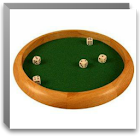Rapid Math Tricks & Tips

All Android applications categories

All Android games categories# Rapid Math Tricks & Tips

752 8.2

8.2 Users
rating

## Screenshots

Description

This Application Demonstrates a slew of time-saving tips and tricks for performing common math calculations. Contains sample for each trick, leading the reader through step-by-step.

These simple math secrets and tricks will forever change how you look at the world of numbers. Get ready to amaze your friends—and yourself—with incredible calculations you never thought you could master.

This Application Shows you how to master difficult problems in addition, subtraction, multiplication, and division quickly, easily and without a calculator. And have fun while doing it!

Features:

1. 675+ Rapid Math Tricks and Tips.
2. Math Tricks and Tips has been categories in Section for ease of use.
3. Step-by-step examples to explain each technique.
4. The application allows the user to Share Quotes with other peoples and friends using Facebook, email, SMS, twitter etc.

Categories :

Application Cover Math Tricks and Tips from Following 8 broad Categories.

1. Divisibility Rules for numbers 3-13
2. Multiplication Tips
3. Finding Percentages
4. Squaring Numbers
5. Multiplying Numbers
6. Dividing Numbers
8. Subtracting Numbers

SAMPLE Math Tricks

Dividing by 9

Answer: Add the digits. If that sum is divisible by nine, then the original number is as well.

Q.) Multiplying by nine

Diana Grinwis says: To multiply by nine on your fingers, hold up ten fingers - if the problem is 9 × 8 you just put down your 8 finger and there's your answer: 72. (If the problem is 9 × 7 just put down your 7 finger: 63.)

Tamzo explains this a little differently:

Take the number you are multiplying 9 by and subtract one. That number is the first number in the solution.

Then subtract that number from nine. That number is the second number of the solution.

Examples: 4 * 9 = 36 4-1=3
9-3=6
solution = 36

8 * 9 = 72
8-1=7
9-7=2
solution = 72
5 * 9 = 45
5-1=4
9-4=5
solution = 45
Sergey writes in: Take the one-digit number you are multipling by nine, and insert a zero to its right. Then subtract the original number from it. For example: if the problem is 9 * 6, insert a zero to the right of the six, then subtract six:
9 * 6 = 60 - 6 = 54

Tags: 6 step math trick , como obtener a en rapid math , gratis math rapid , examples of mathematical tricks , rapid math question for 6 , , rapid math tricks , rapid math tricks & tips descargar , rapid math tricks and tips

from 752 reviews

"Great"

8.2